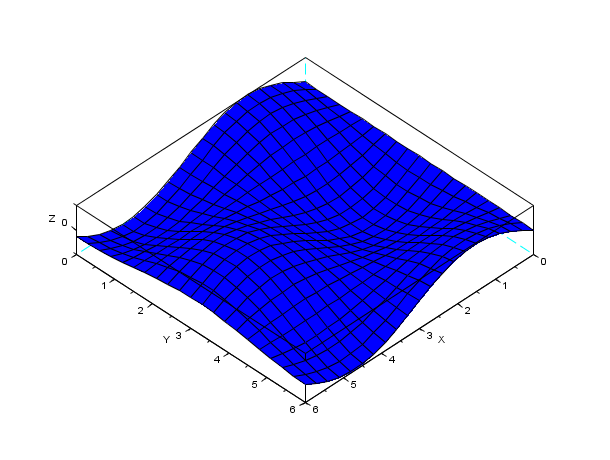Scilab Home page | Wiki | Bug tracker | Forge | Mailing list archives | ATOMS | File exchange
Change language to: English - Français - Português - 日本語 -

See the recommended documentation of this function

Справка Scilab >> Графики > 3d_plot > genfac3d

# genfac3d

Compute facets of a 3D surface

### Syntax

`[xx,yy,zz]=genfac3d(x,y,z,[mask])`

### Arguments

xx,yy,zz

matrices of size (4,n-1xm-1). `xx(:,i)` ,`yy(:,i)` and `zz(:,i)` are respectively the x-axis, y-axis and z-axis coordinates of the 4 points of the ith four sided facet.

x

x-axis coordinates vector of size m.

y

y-axis coordinates vector of size n.

z

matrix of size (m,n). `z(i,j)` is the value of the surface at the point (x(i),y(j)).

boolean optional matrix with same size as `z` used to select the entries of `z` to be represented by facets.

### Description

`genfac3d` computes a four sided facets representation of a 3D surface `z=f(x,y)` defined by `x`, `y` and `z`.

### Examples

```t=[0:0.3:2*%pi]';
z=sin(t)*cos(t');
[xx,yy,zz]=genfac3d(t,t,z);
plot3d(xx,yy,zz)```